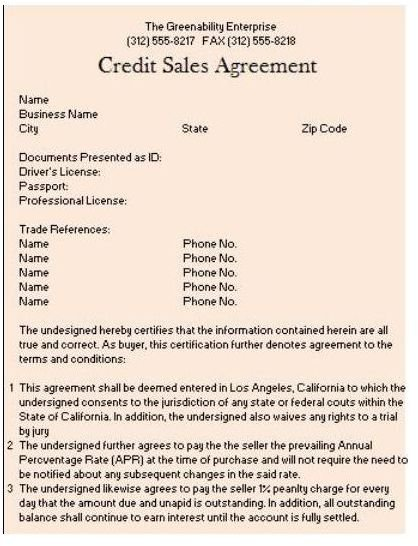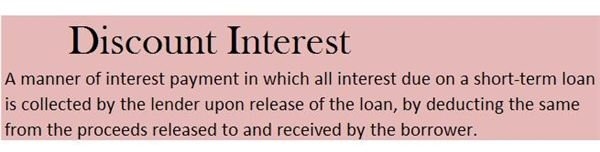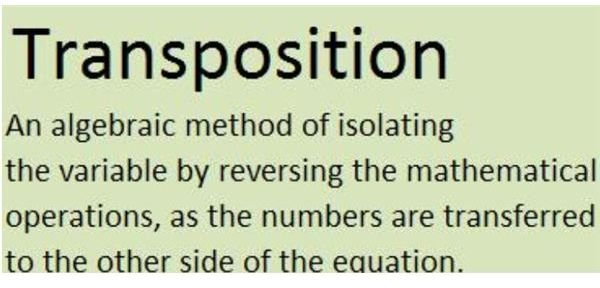# Determine Interest Rates on Notes Receivable

Page content

## Different Documents as Resources for Data on Receivables

A receivable represents an amount loaned out, or a purchase extended to a customer on credit. It is payable on a specific date and usually earns interest from the date the transaction transpired through the date the amount becomes due. The due date is technically referred to as the maturity date.An amount loaned may be described on the balance sheet statement as notes receivable. This would connote that the amount was distinctively granted as a regular loan. As such, the transaction is always accompanied by a promissory note (see image on your left), which states the principal amount, the date of granting, the maturity date, the term or duration of the loan and the applicable interest rate.

It is also important for a business to distinguish a receivable account as trade or non-trade in order to readily determine the aggregate amount of credit sales from those that are not. Most often, a purchase on credit is accompanied by a “Credit Sales Agreement” (see image on your right). This document embodies the principal amount and the terms, including the rate of interest often referred to as “annual percentage rate” (APR).

## Calculating the Amount of InterestTo illustrate how interest is calculated, we will use the following givens:

Date Released: May 15, 2011

Principal Amount - \$20,000

Term: six (6) months or 180 days

Discount Interest Rate: 5% p.a.( see image for definition of discount interest)

• To calculate the amount of interest due, we use the basic formula = Principal x Rate x Time or PRT.

Interest = \$20,000 x 5% x 180/360

= \$20,000 x .05 x 180/360

= \$1,000 x 180/360

Interest = \$500

Note: If the term of the loan is for less than a year, the standard procedure is to divide the interest by 360 days or 12 months, since the rate is stated on a per-year or per-annum basis.

• To calculate the net proceeds of the loan, we simply deduct the interest from the principal amount:

Net Proceeds = \$20,000 - \$500 = \$19,500

## Transposing a Formula to Determine an Unknown

However, there will be instances that a borrower does not have the accompanying promissory note at hand. Yet he or she would like to know the interest rate used as the basis for calculating the net proceeds. Typically, the only information available is the net proceeds and the term of the loan.

In order to determine the interest rate on the note receivable, all one has to do is to perform the calculation by doing the opposite of the basic formula PRT. This simply means that if the mathematical operation was originally performed by way of multiplication, then do the opposite mathematical operation, which is division.

• The first step is to determine the principal amount. In this example, the formula “Net Proceeds = Principal - Interest” (\$19,500 = P - \$500) will be transposed as:

\$19,500 + \$500 = P

\$20,000 = P or Principal• After establishing the principal amount, proceed with the interest rate calculation by observing the same method of transferring or transposing the basic PRT formula on the left side. This is to denote that all mathematical operations are to be reversed. Make it a point to leave the unkown on the right side.

Interest = Principal x Rate x Time

\$500 = \$20,000 x Rate x 180/360

(\$500 ÷ \$20,000) x 360 ÷180 = Rate

0.025 x 360 ÷180 = Rate

.05 x 100% = Rate

5% = Rate

As a note, however, understand that the amount to be paid on the due date is still the principal amount of \$20,000 and not the net proceeds. The lender merely collects the interest due in advance by deducting it from the proceeds.

## Calculating the Interest Based on Diminishing Balance

Some loans, however, are payable on a monthly basis. This means that the principal amount of the loan will be reduced each month — thus the interest rate cannot be calculated on a straight-line basis. Instead, computations shall be performed after establishing the diminished balance of the loan.

To calculate the interest for the first month, the value used as principal is still the original loan amount, which is \$20,000 based on the givens. The interest, however, is calculated on a per-month basis, and the base year used is 365, since the formula will now use the exact number of days.

For this example, the \$20,000 principal is to be divided into six monthly installments, which is equivalent to \$3,333.33 per month.

(1) Interest for the first month (May 15 to June 15) = \$20,000 x 5% x 32/360

= \$1,000 x 32/365

Interest for the 1st month = \$87.67

(2) Interest for the second month (June 16 to July 15) = (\$20,000 - \$3,333.33) x 5% x 30/365

Interest for the 2nd month = \$16,666.67 x 5% x 30/365

= \$833.33 x 30/365

Interest for the 2nd month = \$68.49

## Transposing the Formula to Determine the Interest Rate Based on Diminishing Balance

Similarly, if a borrower does not know the interest rate and the only information available is the monthly amortization, simply perform the reverse of the mathematical operations in order to determine the interest rate on the notes receivable. Again, you have to transpose the “Interest = PRT” formula and leave the symbol for the unknown on the right side.

\$68.49 = \$16, 666.67 x R x 30/365

(\$68.49 ÷ \$16, 666.67) x 365 ÷ 30 = R

0.0041 x 365 ÷ 30 = R

0.0499 Or 5 x 100% = R

5% = R or Rate

Take note that the amount used as principal changes every time an amortization payment is made.

Most loans paid on an installment basis are usually provided with a monthly amortization schedule to serve as a payment reference. Nevertheless, you can learn how to prepare this kind of schedule by using the methods presented above. For this purpose, you can use as a guideline the information furnished in a separate article entitled “Creating a Loan Amortization Table Using Excel”.

## Reference and Image Credit Section

Reference:

• All explanations were based on the author’s expertise as a former bank internal auditor.

Image Credit:

• Sample promisory note, credit sales agreement and screenshot image of definitions were created by the author for this article.